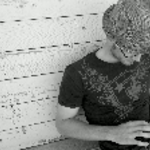Community Profile# Jered Wells

### Landauer Medical Physics

Last seen: 2 months ago Active since 2012

Radiation physicist at Landauer Medical Physics specializing in medical image processing, radiation dosimetry, and medical image quality and metrology. Other specialties include noise/dose reduction, image segmentation, and algorithm development.

#### Statistics

All
•••••#### Content Feed

View by

Submitted

lorentzfit(x,y,varargin)
LORENTZFIT fits a single- or multi-parameter Lorentzian function to dataSolved

How to subtract?
* Imagine you need to subtract a few numbers using MATLAB. * You will not be using eval for this task. * Given two ASCII strin...

5 years ago

Solved

Make a checkerboard matrix
Given an integer n, make an n-by-n matrix made up of alternating ones and zeros as shown below. The a(1,1) should be 1. Examp...

5 years ago

Solved

Find all elements less than 0 or greater than 10 and replace them with NaN
Given an input vector x, find all elements of x less than 0 or greater than 10 and replace them with NaN. Example: Input ...

5 years ago

Solved

Triangle Numbers
Triangle numbers are the sums of successive integers. So 6 is a triangle number because 6 = 1 + 2 + 3 which can be displa...

5 years ago

Solved

Column Removal
Remove the nth column from input matrix A and return the resulting matrix in output B. So if A = [1 2 3; 4 5 6]; and ...

5 years ago

Solved

Select every other element of a vector
Write a function which returns every other element of the vector passed in. That is, it returns the all odd-numbered elements, s...

5 years ago

Question

How to use neural network to perform image segmentation?
I have a clinical need to perform lung segmentation on AP and PA chest radiographs. Using conventional segmentation techniques (...

5 years ago | 1 answer | 0

### 1

Submitted

nortonbeer(W,varargin)
NORTONBEER produces a one-dimensional Norton-Beer apodization filter

Question

Why does MATLAB resample display data for *some* large images and not others? Can this be turned off?
When displaying large images (i.e. images with dimension greater than the screen resolution) using IMSHOW, IMAGESC, and similar ...

6 years ago | 1 answer | 0

### 1

Solved

Pizza!
Given a circular pizza with radius _z_ and thickness _a_, return the pizza's volume. [ _z_ is first input argument.] Non-scor...

6 years ago

Solved

Determine if input is odd
Given the input n, return true if n is odd or false if n is even.

6 years ago

Solved

Given a and b, return the sum a+b in c.

6 years ago

Solved

Find the sum of all the numbers of the input vector
Find the sum of all the numbers of the input vector x. Examples: Input x = [1 2 3 5] Output y is 11 Input x ...

6 years ago

Solved

Make the vector [1 2 3 4 5 6 7 8 9 10]
In MATLAB, you create a vector by enclosing the elements in square brackets like so: x = [1 2 3 4] Commas are optional, s...

6 years ago

Solved

Times 2 - START HERE
Try out this test problem first. Given the variable x as your input, multiply it by two and put the result in y. Examples:...

6 years ago

Submitted

Grayscale Canny Edge Detection
Performs Canny edge detection with grayscale magnitude and direction outputsQuestion

Can I run a "clear java" command but still keep a variable in the workspace?
Hi: I am trying to run "clear java" after temporarily modifying some files in the dynamic Java path. This also clears all var...

8 years ago | 2 answers | 0

### 2

Question

MATLAB's dicominfo function sometimes misreads DICOM header data. I'd like to automatically call ImageJ from the MATLAB command ...

8 years ago | 1 answer | 0

### 1

Submitted

dicomLUT
DICOMLUT applies an embedded lookup table to a DICOM image

Submitted

Center of Mass
CENTEROFMASS finds the center of mass of the N-dimensional input array

Submitted

Point cloud normal vector
NORMND computes the vector normal to points in an N-D point cloudSubmitted

Simpson's 1/3 and 3/8 rules
SIMPSON: Simpson's rule for quadratic and cubic numerical integration

Submitted

Stem and leaf plot
This is a simple utility for making stem and leaf plots output to the command window.

Submitted

R-square: The coefficient of determination
RSQUARE is a simple routine for computing R-square (coefficient of determination).